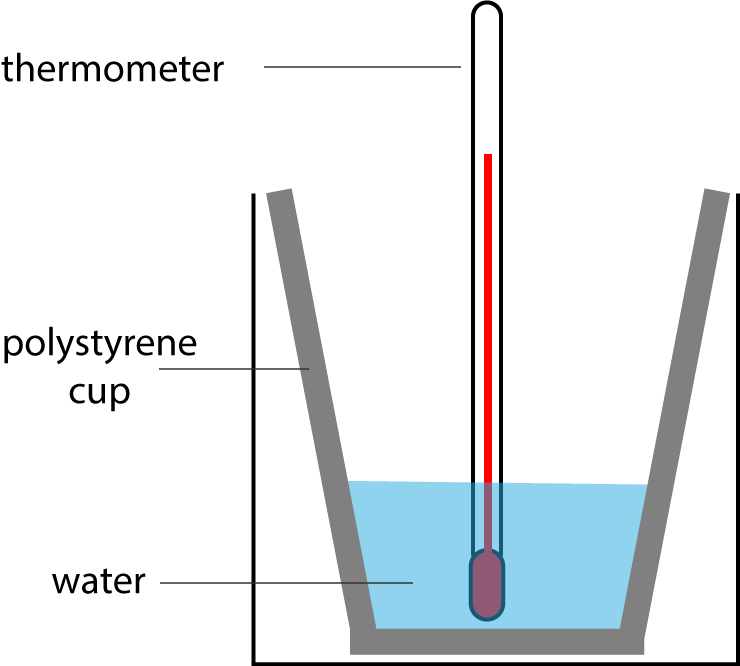Chemistry Edexcel GCSE:
Energy Changes

Page 1

1. Which of the following reactions is exothermic?

 Initial temperature / °C Final temperature / °C A 24 19 B 21 23 C 15 3 D 10 -2
2. How do the temperature and energy change in an endothermic reaction?
• A.   temperature decreases and energy is taken in from surroundings
• B.   temperature decreases and energy is given out to surroundings
• C.   temperature increases and energy is taken in from surroundings
• D.   temperature increases and energy is given out to surroundings
3. A student reacted 25cm3 of sodium hydroxide solution with 25cm3 of dilute sulfuric acid. There was no visible change but he observed that the temperature rose by 3°C.

What types of reaction are taking place?

• A.   exothermic and displacement
• B.   exothermic and neutralisation
• C.   endothermic and displacement
• D.   endothermic and neutralisation
4. Which of the following changes of state is exothermic?
• A.   Fe(s)  →  Fe(l)
• B.   H2O(l)  →  H2O(g)
• C.   NH3(g)  →  NH3(l)
• D.   I2(s)  →  I2(g)

Q5-6:
A student investigated the reactivity of four metals W, X, Y and Z by reacting them with copper(II)sulfate solution. The temperature of the solution was recorded before and after reaction:

 Metal Initial temperature / °C Final temperature / °C W 24.3 37.6 X 24.3 24.3 Y 24.3 26.2 Z 24.3 35.9

5. The order of reactivity of the metals , from most to least reactive is:
• A.   W, X, Y, Z
• B.   W, Z, Y, X
• C.   X, Y, Z, W
• D.   Z, Y, X, W
6. Which of the following did the student NOT need to keep the same in each experiment to ensure a fair test?
• A.  the  same volume of copper(II)sulfate solution
• B.   the same concentration of copper(II)sulfate solution
• C.   the same number of moles of each metal
• D.   the same thermometer

7. Complete the following sentence:

The overall heat energy change for a reaction is endothermic if heat energy is released in forming bonds in the products than is required in breaking bonds in the reactants.

8. Which of the following is true about the temperature and the sign of the energy change, for an exothermic reaction?

 Temperature Sign of energy change A decreases negative B decreases positive C increases negative D increases positive

Q9-10:
A student used the following apparatus to measure the temperature change when ammonium nitrate dissolved in water. He noticed that the temperature dropped.He used 0.024 moles of solid and calculated that the heat change was 1200 J

9. Why did the student use a polystyrene cup rather than a beaker for this experiment?
• A.   polystyrene is cheaper than a beaker
• B.   polystyrene is an insulator
• C.   polystyrene does not break if dropped
• D.   polystyrene does not react with ammonium nitrate
10. The activation energy for a reaction is..
• A. the minimum energy required by product particles for a reaction to occur
• B. the minimum energy required by reactant particles for a reaction to occur
• C. the difference in energy between reactants and products
• D. the energy supplied to the reactants during a reaction